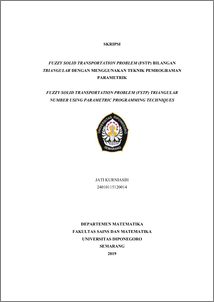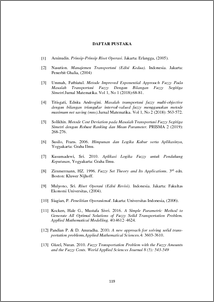# FUZZY SOLID TRANSPORTATION PROBLEM (FSTP) TRIANGULAR NUMBER USING PARAMETRIC PROGRAMMING TECHNIQUES

KURNIASIH, JATI (2019) FUZZY SOLID TRANSPORTATION PROBLEM (FSTP) TRIANGULAR NUMBER USING PARAMETRIC PROGRAMMING TECHNIQUES. Undergraduate thesis, UNDIP.Preview
PDF
155KbPDF
Restricted to Repository staff only

737KbPreview
PDF
85Kb

## Abstract

Fuzzy solid transportation problem (FSTP) has fuzzy cost coefficient, fuzzy supply, fuzzy demand and fuzzy conveyance with triangular numbers. FSTP optimal solutions there are three stages: the first stage to determine the maximum feasibility level based on the amount of supply, demand, and conveyance, the second stage to get breaking points from the fuzzy cost coefficient, and the third stage to build the FSTP optimal solution using parametric programming techniques. Parametric programming technique has 5 steps: the zero step to initialization index value, the first step to find the objective function value, the second step to solve the problem with an additional program, the third step checks the optimal solution to satisfy the feasibility conditions, and the fourth step if the optimal solution does not satisfy the feasibility conditions. Keywords: Fuzzy solid transportation problem (FSTP), triangular number, parametric programming techniques.

Item Type: Thesis (Undergraduate) Q Science > QA Mathematics Faculty of Science and Mathematics > Department of Mathematics 84252 INVALID USER 13 Jun 2022 09:05 13 Jun 2022 09:05

Repository Staff Only: item control page